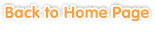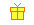Sample questions are shown below. Just click to start!• Chapter 1
Real Numbers
••
Cube Roots and Real Numbers• Chapter 2
Linear Functions
•
Variables and Functions•• Chapter 3
Multiplying and Dividing Expressions
•
Multiplying Expressions••
Dividing Expressions•
Factorization• Chapter 4
Rational Expressions
•
Operations with Rational Expressions••
Inverse Variation• Chapter 5• Chapter 6
Symmetry
•
Axially symmetric figures••
Rotation and Rotaional Symmetry• Chapter 7• Chapter 8